### Home > MC1 > Chapter 6 > Lesson 6.2.4 > Problem6-83

6-83.
1. . Convert each mixed number to a fraction greater than one, or each fraction greater than one to a mixed number. Homework Help ✎

1. 5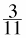2.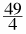3. 3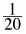4.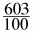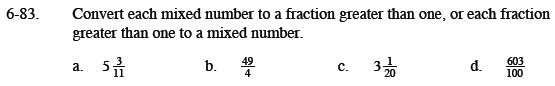See the Math Notes box below to help you convert each number.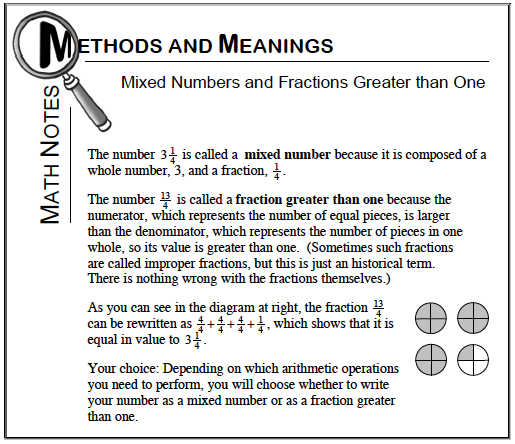$12\frac{1}{4}$

For parts (c) and (d), refer to the Math Notes box.